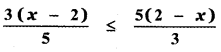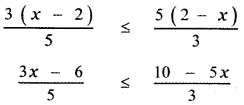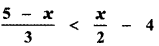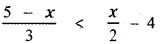Tamilnadu State Board New Syllabus Samacheer Kalvi 11th Maths Guide Pdf Chapter 2 Basic Algebra Ex 2.3 Text Book Back Questions and Answers, Notes.

## Tamilnadu Samacheer Kalvi 11th Maths Solutions Chapter 2 Basic Algebra Ex 2.3

Question 1.
Represent the following inequalities in the interval notation:
(i) x ≥ – 1 and x < 4
x ≥ – 1 and x < 4
x ∈ [- 1, 4)(ii) x ≤ 5 and x ≥ – 3
x ≤ 5 and x ≥ – 3
– 3 ≤ x ≤ 5
∴ x ∈ [- 3, 5 ]

(iii) x < – 1 or x < 3
x < – 1 or x < 3
x ∈ (-∞, 3)

(iv) -2x > 0 or 3x – 4 < 11
– 2x > 0 or 3x – 4 < 11
2x < 0 or 3x < 11 + 4
x < 0 or x < $$\frac{15}{3}$$
x < 0 or x < 5
x ∈ (- ∞, 5)Question 2.
Solve 23x < 100 when
(i) x is a natural number,
(ii) x is an integer.
Given 23x < 100
(i) When x is a natural number
23x < 100
⇒ x < $$\frac{100}{23}$$
⇒ x < 4.347
⇒ x = 1, 2, 3, 4
∴ The solution set is { 1, 2, 3 , 4 }

(ii) When x is an integer
23x < 100
⇒ x < $$\frac{100}{23}$$
⇒ x < 4.347
⇒ x = …… , – 3, – 2, – 1, 0, 1, 2, 3, 4
Hence the solution set is
{ ………, – 3, – 2, – 1, 0, 1 , 2 , 3 , 4 }Question 3.
Solve – 2x ≥ 9 when
(i) x is a real number,
(ii) x is an integer
(iii) x is a natural number.
Given – 2x ≥ 9
⇒ – x ≥ $$\frac{9}{2}$$
⇒ x ≤ $$-\frac{9}{2}$$

(i) When x is a real number
x ≤ $$-\frac{9}{2}$$
The solution set is $$\left(-\infty,-\frac{9}{2}\right]$$

(ii) x is an integer
x ≤ –$$-\frac{9}{2}$$
x ≤ – 4.5
x = …………., -7, – 6, -5

(iii) x is a natural number
x ≤ – $$\frac{9}{2}$$
x ≤ – 4.5
Since there exists no natural number less than – $$\frac{9}{2}$$
∴ No solutionQuestion 4.
Solve:
(i)3 (3x – 6) ≤ 5 (10 – 5x)
9x – 18 ≤ 50 – 25 x
9x + 25 x ≤ 50 + 18
34x ≤ 68
x ≤ $$\frac{68}{34}$$ = 2
x ≤ 2
∴ The solution set is (-∞, 2](ii)Multiplying both sides by 3, we have
5 – x < $$\frac{3 x}{2}$$ – 12
Multiplying both sides by 2, we have
10 – 2x < 3x – 24
10 + 24 < 3x + 2x
34 < 5x
$$\frac{34}{5}$$ < x
x > $$\frac{34}{5}$$
∴ The solution set is $$\left(\frac{34}{5}, \infty\right)$$Question 5.
To secure an A grade, one must obtain an average of 90 marks or more in 5 subjects each of a maximum of 100 marks. If one scored 84, 87, 95, 91 in the first four subjects, what is the minimum mark one scored in the fifth subject to get an A grade in the course?
Required marks = 5 × 90 = 450
Total marks obtained in 4 subjects = 84 + 87 + 95 + 91 = 357
So required marks in the fifth subject = 450 – 357 = 93

Question 6.
A manufacturer has 600 litres of a 12 percent solution of acid. How many litres of a 30 percent acid solution must be added to it so that the acid content in the resulting mixture will be more than 15 percent but less than 18 percent?
Amount of 12% solution of acid = 600 litres
Let x be the required number litres of 30 % acid solution to be added to the given 600 litres of 12 % acid solution to make the resulting mixture will be more than 15 % but less than 18 %.

∴ Total amount of mixture = (600 + x) litres
30% acid solution of x litres + 12% acid solution of 600 litres > 15% acid solution of (600 + x) litres
$$\frac{30}{100}$$ × x + $$\frac{12}{100}$$ × 600 > $$\frac{15}{100}$$ × (600 + x)
30x + 7200 > 9000 + 15x
30x – 15x > 9000 – 7200
15x > 1800
x > $$\frac{1800}{15}$$ = 120
x > 120 ——– (1)
Also 30% acid solution of x litres + 12% acid solution of 600 litres < 18% acid solution of ( 600 + x) litres.
$$\frac{30}{100}$$ × x + $$\frac{12}{100}$$ × 600 < $$\frac{15}{100}$$ × (600 + x)
30x + 7200 < 18 (600 + x)
30x + 7200 < 10800 + 18x
30x – 18x < 10,800 – 7200
12x < 3600
x < $$\frac{3600}{12}$$ = 300
x < 300 ——- (2)
From equations (1) and (2), we get ) 120 < x < 300
∴ The numbers of litres of the 30% acid solution to be added is greater than 120 litres and less than 300 litres.Question 7.
Find all pairs of consecutive odd natural numbers both of which are larger than 10 and their sum is less than 40.
Let x and x + 2 be the two pair of consecutive odd natural numbers.
Given x > 10 ——— (1)
and x + 2 > 10 ——— (2)
Also x + (x + 2) < 40 ——— (3)
From equations (1), we have
x = 11, 13 , 15, 17, 19, 21 …………
Using equation (3), the required pairs are
(11, 13), (13, 15), (15, 17), ( 17, 19), (19 ,21 ) is not possible since 19 + 21 = 40

Question 8.
A model rocket is launched from the ground. The height h reached by the rocket after t seconds from lift off is given by h(t) = – 5t2 + 100t, 0 ≤ t ≤ 20. At what times, the rocket is 495 feet above the ground?
h(t) = -5t2 + 100t
at t = 0, h(0) = 0
at t = 1, h(1) = -5 + 100 = 95
at t = 2, h(2) = -20 + 200 = 180
at t =3, h(3) = -45 + 300 = 255
at t = 4, h(4) = -80 + 400 = 320
at t = 5, h(5) = -125 + 500 = 375
at t = 6, h(6) = – 180 + 600 = 420
at t = 7, h(7) = -245 + 700 = 455
at t = 8, h(8) = – 320 + 800 = 480
at t = 9, h(9) = -405 + 900 = 495
So, at 9 secs, the rocket is 495 feet above the ground.Question 9.
A plumber can be paid according to the following schemes: In the first scheme he will be paid rupees 500 plus rupees 70 per hour and in the second scheme, he will pay rupees 120 per hour. If he works x hours, then for what value of x does the first scheme give better wages?
I scheme with x hr
500 + (x- 1) 70 = 500 + 70x – 70 = 430 + 70x
II scheme with x hours
120x
Here I > II
⇒ 430 + 70x > 120x
⇒ 120x – 70x < 430
50x < 430
$$\frac{50 x}{50}<\frac{430}{50}$$
x < 8.6 (i.e.) when x is less than 9 hrs the first scheme gives better wages.Question 10.
A and B are working on similar jobs but their monthly salaries differ by more than Rs. 6000. If B earns rupees 27,000 per month, then what are the possibilities of A’s salary per month?